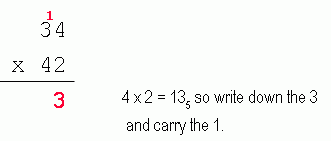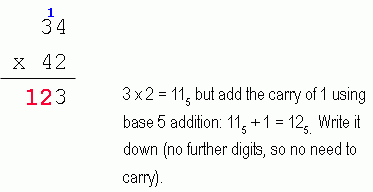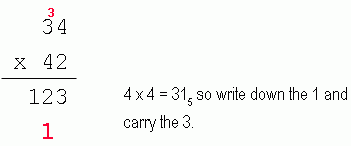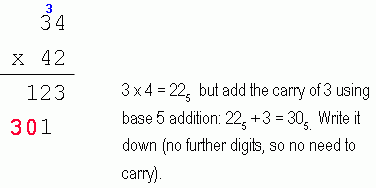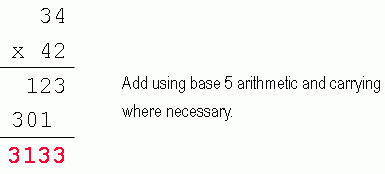SEARCH HOMEMath Central Quandaries & QueriesQuestion from Imaiya, a student: Hi Math Central! First off, I'd like to applaud you on your awesome site! =) I regularly come here for math help, or revision in general. In class we've been learning about numbers with a base other than ten. For example, base 7, base 5, and base 6. What's really stumping me is how to multiply these numbers, when they aren't a base of 10. I've had it explained to me once, but don't seem to understand it. Here are the equations, and I've listed the first few numbers for that particular base. Base 5: 0,1,2,3,4,10,11,12,13,14,20 etc... The Equation: 34                                                                              x 42 Base 9: 1,2,3,4,5,6,7,8,10,11,12,13,14, etc... The Equation: 56                                                                                   x 78 If you could explain to me how to multiply numbers with any type of base, I'd really appreaciate it. Also if you could show me how to do one of the questions that I listed, that would really help me =) Thanks so much, and once again, thank you for your great site!Hi Imaiya,

We're glad to help! It's always encouraging to hear that our site is useful for people and if you have ideas or suggestions for the site, let us know by emailing us at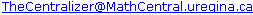.

Each digit represents another power of the base. So think about base 10 numbers. Reading leftwards from the decimal, we have places that represent 100, 101, 102, 103, etc. You can see that 2007 is composed of 2 x 10+ 0 x 10+ 0 x 10+ 7 x 100.

Now let's think about base 8. If I have the base 8 number 3708 (we use a subscript after a number to show that it is to be interpreted in that base rather than the normal base 10) then I know that the places (again leftwards from the decimal) denote 80, 81, 82, etc. So 370= 3 x 82 + 7  x 81 + 0 x 80.

When we multiply numbers in other bases, we can do it two ways:

First method: Convert both numbers to base 10, multiply them normally, then convert that back to the desired base. This is usually preferable when multiplying numbers of different bases.

Here's an example: multiply 4556 by 7059 and express the product in base 4.

• Convert to base 10:
4556 = 4 x 62 + 5 x 61 + 5 x 60 = 144 + 30 + 5 = 179.
7059 = 7 x 92 + 0 x 91 + 5 x 90 = 567 + 5 = 572.
• Multiply normally:
179 x 572 = 102388.
• Convert to base 4:
102388 / 4 = 25597 remainder 0.
25597 / 4 = 6399 remander 1.
6399 / 4 = 1599 remainder 3.
1599 / 4 = 399 remainder 3.
399 / 4 = 99 remainder 3.
99 / 4 = 24 remainder 3.
24 / 4 = 6 remainder 0.
6 / 4 = 1 remainder 2.
1 / 4 = 0 remainder 1.

Second method: Build a times table for the base you are using. This only works if the two factors are the same base.

For example, your first question was to solve 345 x 425:

 Base 5 times table 0 1 2 3 4 0 0 1 0 1 2 0 2 4 3 0 3 11 14 4 0 4 13 22 31

Now multiply it as you would with base 10, but using this times table. Remember to use base 5 when carrying as well: